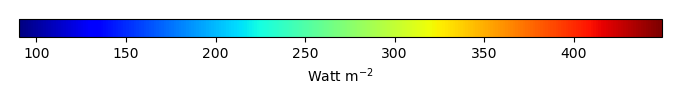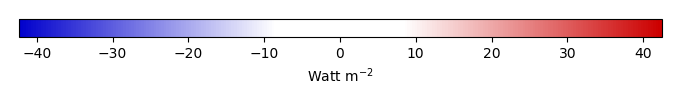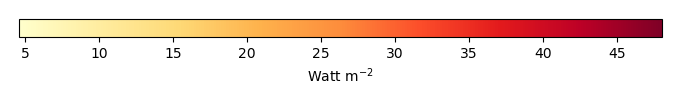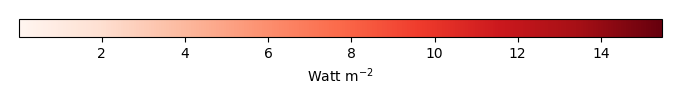# Mean State

Period Mean (original grids) [Watt m-2]
Model Period Mean (intersection) [Watt m-2]
Model Period Mean (complement) [Watt m-2]
Benchmark Period Mean (intersection) [Watt m-2]
Benchmark Period Mean (complement) [Watt m-2]
Bias [Watt m-2]
RMSE [Watt m-2]
Phase Shift [months]
Bias Score 
RMSE Score 
Seasonal Cycle Score 
Spatial Distribution Score 
Interannual Variability Score 
Overall Score 
Benchmark [-] 397.
CRUNCEPv7 [-] 406. 406. 0.00 396. 408. 11.3 16.6 0.567 0.33 0.40 0.94 0.76 0.76 0.60
GSWP3v1 [-] 395. 395. 0.00 396. 408. -0.219 6.93 0.212 0.82 0.50 0.97 1.0 0.75 0.76
WFDEI [-] 417. 418. 0.00 396. 408. 22.7 28.2 0.840 0.17 0.30 0.91 0.61 0.65 0.49
Period Mean (original grids) [Watt m-2]
Model Period Mean (intersection) [Watt m-2]
Model Period Mean (complement) [Watt m-2]
Benchmark Period Mean (intersection) [Watt m-2]
Benchmark Period Mean (complement) [Watt m-2]
Bias [Watt m-2]
RMSE [Watt m-2]
Phase Shift [months]
Bias Score 
RMSE Score 
Seasonal Cycle Score 
Spatial Distribution Score 
Interannual Variability Score 
Overall Score 
Benchmark [-] 384.
CRUNCEPv7 [-] 395. 394. 0.00 384. 397. 10.5 17.7 0.930 0.61 0.63 0.89 0.83 0.74 0.72
GSWP3v1 [-] 384. 384. 0.00 384. 397. -0.0965 9.48 0.181 0.90 0.69 0.99 1.0 0.91 0.86
WFDEI [-] 402. 402. 0.00 384. 397. 17.8 28.6 0.919 0.50 0.46 0.89 0.75 0.73 0.63
Period Mean (original grids) [Watt m-2]
Model Period Mean (intersection) [Watt m-2]
Model Period Mean (complement) [Watt m-2]
Benchmark Period Mean (intersection) [Watt m-2]
Benchmark Period Mean (complement) [Watt m-2]
Bias [Watt m-2]
RMSE [Watt m-2]
Phase Shift [months]
Bias Score 
RMSE Score 
Seasonal Cycle Score 
Spatial Distribution Score 
Interannual Variability Score 
Overall Score 
Benchmark [-] 362.
CRUNCEPv7 [-] 369. 368. 0.00 361. 382. 9.69 17.7 1.20 0.62 0.62 0.84 0.97 0.71 0.73
GSWP3v1 [-] 359. 359. 0.00 361. 382. 0.0495 8.96 0.237 0.88 0.70 0.97 1.0 0.85 0.85
WFDEI [-] 368. 368. 0.00 361. 382. 8.67 19.2 1.12 0.62 0.57 0.87 0.93 0.83 0.73
Period Mean (original grids) [Watt m-2]
Model Period Mean (intersection) [Watt m-2]
Model Period Mean (complement) [Watt m-2]
Benchmark Period Mean (intersection) [Watt m-2]
Benchmark Period Mean (complement) [Watt m-2]
Bias [Watt m-2]
RMSE [Watt m-2]
Phase Shift [months]
Bias Score 
RMSE Score 
Seasonal Cycle Score 
Spatial Distribution Score 
Interannual Variability Score 
Overall Score 
Benchmark [-] 239.
CRUNCEPv7 [-] 205. 205. 0.00 238. 251. -30.9 33.7 0.0673 0.55 0.78 1.0 0.97 0.74 0.80
GSWP3v1 [-] 236. 236. 0.00 238. 251. 0.254 9.46 0.0140 0.96 0.83 1.0 1.0 0.89 0.92
WFDEI [-] 206. 206. 0.00 238. 251. -30.0 34.6 0.0562 0.56 0.72 1.0 0.99 0.76 0.79
Period Mean (original grids) [Watt m-2]
Model Period Mean (intersection) [Watt m-2]
Model Period Mean (complement) [Watt m-2]
Benchmark Period Mean (intersection) [Watt m-2]
Benchmark Period Mean (complement) [Watt m-2]
Bias [Watt m-2]
RMSE [Watt m-2]
Phase Shift [months]
Bias Score 
RMSE Score 
Seasonal Cycle Score 
Spatial Distribution Score 
Interannual Variability Score 
Overall Score 
Benchmark [-] 411.
CRUNCEPv7 [-] 427. 427. 0.00 410. 415. 19.7 22.9 1.86 0.070 0.33 0.72 0.57 0.70 0.45
GSWP3v1 [-] 408. 408. 0.00 410. 415. 0.0344 5.44 0.531 0.67 0.40 0.92 0.99 0.74 0.69
WFDEI [-] 438. 438. 0.00 410. 415. 28.7 30.2 1.77 0.048 0.22 0.73 0.72 0.59 0.42
Period Mean (original grids) [Watt m-2]
Model Period Mean (intersection) [Watt m-2]
Model Period Mean (complement) [Watt m-2]
Benchmark Period Mean (intersection) [Watt m-2]
Benchmark Period Mean (complement) [Watt m-2]
Bias [Watt m-2]
RMSE [Watt m-2]
Phase Shift [months]
Bias Score 
RMSE Score 
Seasonal Cycle Score 
Spatial Distribution Score 
Interannual Variability Score 
Overall Score 
Benchmark [-] 264.
CRUNCEPv7 [-] 243. 243. 0.00 264. 277. -20.2 22.6 0.0306 0.70 0.84 1.0 0.98 0.86 0.87
GSWP3v1 [-] 264. 263. 0.00 264. 277. 0.0194 8.45 0.00704 0.97 0.86 1.0 1.0 0.81 0.92
WFDEI [-] 243. 243. 0.00 264. 277. -20.9 25.9 0.0255 0.69 0.76 1.0 0.99 0.87 0.85
Period Mean (original grids) [Watt m-2]
Model Period Mean (intersection) [Watt m-2]
Model Period Mean (complement) [Watt m-2]
Benchmark Period Mean (intersection) [Watt m-2]
Benchmark Period Mean (complement) [Watt m-2]
Bias [Watt m-2]
RMSE [Watt m-2]
Phase Shift [months]
Bias Score 
RMSE Score 
Seasonal Cycle Score 
Spatial Distribution Score 
Interannual Variability Score 
Overall Score 
Benchmark [-] 294.
CRUNCEPv7 [-] 279. 278. 0.00 293. 336. -11.9 19.7 0.0697 0.76 0.78 0.99 0.99 0.83 0.85
GSWP3v1 [-] 291. 291. 0.00 293. 336. 0.277 9.99 0.0242 0.94 0.82 1.0 1.0 0.78 0.90
WFDEI [-] 280. 279. 0.00 293. 336. -10.8 21.6 0.101 0.76 0.73 0.99 1.0 0.84 0.84
Period Mean (original grids) [Watt m-2]
Model Period Mean (intersection) [Watt m-2]
Model Period Mean (complement) [Watt m-2]
Benchmark Period Mean (intersection) [Watt m-2]
Benchmark Period Mean (complement) [Watt m-2]
Bias [Watt m-2]
RMSE [Watt m-2]
Phase Shift [months]
Bias Score 
RMSE Score 
Seasonal Cycle Score 
Spatial Distribution Score 
Interannual Variability Score 
Overall Score 
Benchmark [-] 379.
CRUNCEPv7 [-] 385. 385. 0.00 377. 410. 14.7 23.2 0.641 0.53 0.67 0.94 0.93 0.74 0.75
GSWP3v1 [-] 371. 372. 0.00 377. 410. -0.454 9.29 0.100 0.88 0.75 0.99 1.0 0.88 0.87
WFDEI [-] 390. 391. 0.00 377. 410. 19.8 27.6 0.682 0.48 0.59 0.93 0.96 0.81 0.72
Period Mean (original grids) [Watt m-2]
Model Period Mean (intersection) [Watt m-2]
Model Period Mean (complement) [Watt m-2]
Benchmark Period Mean (intersection) [Watt m-2]
Benchmark Period Mean (complement) [Watt m-2]
Bias [Watt m-2]
RMSE [Watt m-2]
Phase Shift [months]
Bias Score 
RMSE Score 
Seasonal Cycle Score 
Spatial Distribution Score 
Interannual Variability Score 
Overall Score 
Benchmark [-] 244.
CRUNCEPv7 [-] 214. 215. 0.00 244. 248. -28.8 31.6 0.00253 0.56 0.77 1.0 0.97 0.84 0.82
GSWP3v1 [-] 243. 243. 0.00 244. 248. 0.0310 8.41 0.00100 0.98 0.84 1.0 1.0 0.88 0.92
WFDEI [-] 215. 215. 0.00 244. 248. -28.2 33.6 0.00539 0.56 0.69 1.0 0.99 0.88 0.80
Period Mean (original grids) [Watt m-2]
Model Period Mean (intersection) [Watt m-2]
Model Period Mean (complement) [Watt m-2]
Benchmark Period Mean (intersection) [Watt m-2]
Benchmark Period Mean (complement) [Watt m-2]
Bias [Watt m-2]
RMSE [Watt m-2]
Phase Shift [months]
Bias Score 
RMSE Score 
Seasonal Cycle Score 
Spatial Distribution Score 
Interannual Variability Score 
Overall Score 
Benchmark [-] 351.
CRUNCEPv7 [-] 353. 353. 0.00 350. 359. 3.02 14.9 0.381 0.75 0.72 0.97 0.88 0.71 0.79
GSWP3v1 [-] 350. 350. 0.00 350. 359. 0.0571 7.85 0.104 0.93 0.78 0.99 1.0 0.91 0.90
WFDEI [-] 352. 352. 0.00 350. 359. 1.80 17.8 0.496 0.76 0.62 0.96 0.90 0.84 0.78
Period Mean (original grids) [Watt m-2]
Model Period Mean (intersection) [Watt m-2]
Model Period Mean (complement) [Watt m-2]
Benchmark Period Mean (intersection) [Watt m-2]
Benchmark Period Mean (complement) [Watt m-2]
Bias [Watt m-2]
RMSE [Watt m-2]
Phase Shift [months]
Bias Score 
RMSE Score 
Seasonal Cycle Score 
Spatial Distribution Score 
Interannual Variability Score 
Overall Score 
Benchmark [-] 308.
CRUNCEPv7 [-] 290. 290. 0.00 307. 324. -13.0 18.2 0.220 0.65 0.74 0.99 0.90 0.86 0.81
GSWP3v1 [-] 304. 304. 0.00 307. 324. 0.0546 8.06 0.0712 0.93 0.78 1.0 1.0 0.74 0.87
WFDEI [-] 288. 288. 0.00 307. 324. -15.4 22.3 0.317 0.61 0.64 0.98 0.90 0.90 0.78
Period Mean (original grids) [Watt m-2]
Model Period Mean (intersection) [Watt m-2]
Model Period Mean (complement) [Watt m-2]
Benchmark Period Mean (intersection) [Watt m-2]
Benchmark Period Mean (complement) [Watt m-2]
Bias [Watt m-2]
RMSE [Watt m-2]
Phase Shift [months]
Bias Score 
RMSE Score 
Seasonal Cycle Score 
Spatial Distribution Score 
Interannual Variability Score 
Overall Score 
Benchmark [-] 344.
CRUNCEPv7 [-] 305. 305. 0.00 311. 360. -2.78 21.3 0.550 0.59 0.63 0.93 0.99 0.73 0.75
GSWP3v1 [-] 308. 308. 0.00 311. 360. -0.0963 9.16 0.113 0.89 0.72 0.99 1.0 0.84 0.86
WFDEI [-] 306. 306. 0.00 311. 360. -2.14 24.0 0.579 0.58 0.58 0.93 0.99 0.78 0.74
Period Mean (original grids) [Watt m-2]
Model Period Mean (intersection) [Watt m-2]
Model Period Mean (complement) [Watt m-2]
Benchmark Period Mean (intersection) [Watt m-2]
Benchmark Period Mean (complement) [Watt m-2]
Bias [Watt m-2]
RMSE [Watt m-2]
Phase Shift [months]
Bias Score 
RMSE Score 
Seasonal Cycle Score 
Spatial Distribution Score 
Interannual Variability Score 
Overall Score 
Benchmark [-] 343.
CRUNCEPv7 [-] 350. 350. 0.00 343. 360. 8.96 20.6 0.463 0.70 0.63 0.96 0.93 0.67 0.75
GSWP3v1 [-] 342. 342. 0.00 343. 360. 0.000187 11.3 0.0949 0.92 0.72 0.99 1.0 0.89 0.87
WFDEI [-] 339. 339. 0.00 343. 360. -1.65 19.2 0.513 0.80 0.60 0.95 1.0 0.71 0.78
Period Mean (original grids) [Watt m-2]
Model Period Mean (intersection) [Watt m-2]
Model Period Mean (complement) [Watt m-2]
Benchmark Period Mean (intersection) [Watt m-2]
Benchmark Period Mean (complement) [Watt m-2]
Bias [Watt m-2]
RMSE [Watt m-2]
Phase Shift [months]
Bias Score 
RMSE Score 
Seasonal Cycle Score 
Spatial Distribution Score 
Interannual Variability Score 
Overall Score 
Benchmark [-] 244.
CRUNCEPv7 [-] 216. 216. 0.00 243. 253. -27.1 29.3 0.0689 0.56 0.79 1.0 0.99 0.75 0.81
GSWP3v1 [-] 243. 243. 0.00 243. 253. -0.0103 8.60 0.0247 0.97 0.83 1.0 1.0 0.88 0.92
WFDEI [-] 216. 216. 0.00 243. 253. -27.5 31.6 0.0782 0.55 0.72 0.99 0.98 0.83 0.80
Period Mean (original grids) [Watt m-2]
Model Period Mean (intersection) [Watt m-2]
Model Period Mean (complement) [Watt m-2]
Benchmark Period Mean (intersection) [Watt m-2]
Benchmark Period Mean (complement) [Watt m-2]
Bias [Watt m-2]
RMSE [Watt m-2]
Phase Shift [months]
Bias Score 
RMSE Score 
Seasonal Cycle Score 
Spatial Distribution Score 
Interannual Variability Score 
Overall Score 
Benchmark [-] 374.
CRUNCEPv7 [-] 372. 372. 0.00 369. 398. 13.3 21.8 0.734 0.45 0.59 0.92 0.93 0.77 0.71
GSWP3v1 [-] 362. 362. 0.00 369. 398. 0.0604 9.12 0.254 0.82 0.68 0.97 1.0 0.87 0.84
WFDEI [-] 376. 376. 0.00 369. 398. 15.8 25.2 0.633 0.43 0.52 0.93 0.95 0.80 0.69
Period Mean (original grids) [Watt m-2]
Model Period Mean (intersection) [Watt m-2]
Model Period Mean (complement) [Watt m-2]
Benchmark Period Mean (intersection) [Watt m-2]
Benchmark Period Mean (complement) [Watt m-2]
Bias [Watt m-2]
RMSE [Watt m-2]
Phase Shift [months]
Bias Score 
RMSE Score 
Seasonal Cycle Score 
Spatial Distribution Score 
Interannual Variability Score 
Overall Score 
Benchmark [-] 332.
CRUNCEPv7 [-] 321. 321. 0.00 331. 370. -6.68 20.0 0.0934 0.79 0.68 0.99 0.91 0.89 0.82
GSWP3v1 [-] 328. 328. 0.00 331. 370. -0.0972 7.51 0.0275 0.95 0.84 1.0 1.0 0.84 0.91
WFDEI [-] 319. 319. 0.00 331. 370. -8.96 23.1 0.127 0.78 0.63 0.99 0.93 0.83 0.80
Period Mean (original grids) [Watt m-2]
Model Period Mean (intersection) [Watt m-2]
Model Period Mean (complement) [Watt m-2]
Benchmark Period Mean (intersection) [Watt m-2]
Benchmark Period Mean (complement) [Watt m-2]
Bias [Watt m-2]
RMSE [Watt m-2]
Phase Shift [months]
Bias Score 
RMSE Score 
Seasonal Cycle Score 
Spatial Distribution Score 
Interannual Variability Score 
Overall Score 
Benchmark [-] 277.
CRUNCEPv7 [-] 254. 254. 0.00 276. 298. -20.7 23.2 0.0749 0.62 0.79 0.99 0.99 0.91 0.85
GSWP3v1 [-] 274. 275. 0.00 276. 298. -0.0815 7.66 0.0260 0.95 0.84 1.0 1.0 0.83 0.91
WFDEI [-] 253. 254. 0.00 276. 298. -21.3 26.3 0.0831 0.61 0.70 0.99 1.0 0.87 0.81
Period Mean (original grids) [Watt m-2]
Model Period Mean (intersection) [Watt m-2]
Model Period Mean (complement) [Watt m-2]
Benchmark Period Mean (intersection) [Watt m-2]
Benchmark Period Mean (complement) [Watt m-2]
Bias [Watt m-2]
RMSE [Watt m-2]
Phase Shift [months]
Bias Score 
RMSE Score 
Seasonal Cycle Score 
Spatial Distribution Score 
Interannual Variability Score 
Overall Score 
Benchmark [-] 296.
CRUNCEPv7 [-] 286. 287. 0.00 295. 324. -7.01 15.9 0.214 0.75 0.73 0.99 0.94 0.84 0.83
GSWP3v1 [-] 293. 294. 0.00 295. 324. -0.178 9.51 0.0536 0.91 0.77 1.0 1.0 0.79 0.87
WFDEI [-] 287. 289. 0.00 295. 324. -6.04 17.7 0.241 0.77 0.66 0.98 0.97 0.82 0.81
Period Mean (original grids) [Watt m-2]
Model Period Mean (intersection) [Watt m-2]
Model Period Mean (complement) [Watt m-2]
Benchmark Period Mean (intersection) [Watt m-2]
Benchmark Period Mean (complement) [Watt m-2]
Bias [Watt m-2]
RMSE [Watt m-2]
Phase Shift [months]
Bias Score 
RMSE Score 
Seasonal Cycle Score 
Spatial Distribution Score 
Interannual Variability Score 
Overall Score 
Benchmark [-] 406.
CRUNCEPv7 [-] 424. 424. 0.00 405. 410. 18.6 23.4 2.09 0.15 0.37 0.67 0.72 0.75 0.50
GSWP3v1 [-] 405. 405. 0.00 405. 410. -0.270 6.26 0.342 0.80 0.50 0.95 1.0 0.82 0.76
WFDEI [-] 432. 432. 0.00 405. 410. 27.3 31.7 2.65 0.085 0.30 0.56 0.73 0.54 0.42
Period Mean (original grids) [Watt m-2]
Model Period Mean (intersection) [Watt m-2]
Model Period Mean (complement) [Watt m-2]
Benchmark Period Mean (intersection) [Watt m-2]
Benchmark Period Mean (complement) [Watt m-2]
Bias [Watt m-2]
RMSE [Watt m-2]
Phase Shift [months]
Bias Score 
RMSE Score 
Seasonal Cycle Score 
Spatial Distribution Score 
Interannual Variability Score 
Overall Score 
Benchmark [-] 376.
CRUNCEPv7 [-] 384. 383. 0.00 376. 374. 8.34 16.3 1.28 0.59 0.60 0.81 0.91 0.84 0.72
GSWP3v1 [-] 376. 375. 0.00 376. 374. -0.175 9.06 0.144 0.89 0.66 0.98 1.0 0.84 0.84
WFDEI [-] 386. 385. 0.00 376. 374. 9.58 19.0 1.13 0.59 0.52 0.85 0.93 0.83 0.71
Period Mean (original grids) [Watt m-2]
Model Period Mean (intersection) [Watt m-2]
Model Period Mean (complement) [Watt m-2]
Benchmark Period Mean (intersection) [Watt m-2]
Benchmark Period Mean (complement) [Watt m-2]
Bias [Watt m-2]
RMSE [Watt m-2]
Phase Shift [months]
Bias Score 
RMSE Score 
Seasonal Cycle Score 
Spatial Distribution Score 
Interannual Variability Score 
Overall Score 
Benchmark [-] 307.
CRUNCEPv7 [-] 289. 288. 0.00 303. 340. -11.5 22.3 0.489 0.46 0.57 0.96 0.99 0.70 0.71
GSWP3v1 [-] 301. 301. 0.00 303. 340. 1.13 13.5 0.185 0.72 0.58 0.98 1.0 0.75 0.77
WFDEI [-] 298. 297. 0.00 303. 340. -3.55 21.7 0.545 0.51 0.51 0.95 0.99 0.76 0.71

# Temporally integrated period mean

BENCHMARK MEANMODEL MEANBIASBIAS SCORERMSERMSE SCOREBENCHMARK INTERANNUAL VARIABILITYMODEL INTERANNUAL VARIABILITYINTERANNUAL VARIABILITY SCOREBENCHMARK MAX MONTHMODEL MAX MONTHDIFFERENCE IN MAX MONTHSEASONAL CYCLE SCORESPATIAL TAYLOR DIAGRAMMODEL COLORS# Spatially integrated regional mean

MODEL COLORSREGIONAL MEANANNUAL CYCLEMONTHLY ANOMALYANNUAL CYCLE# All Models

BenchmarkCRUNCEPv7GSWP3v1WFDEI# Data Information

creation_date: Mon Jun 30 23:41:07 PDT 2014

source_file: This product is generated from monthly 1 degree GEWEX SRB Radiation observations